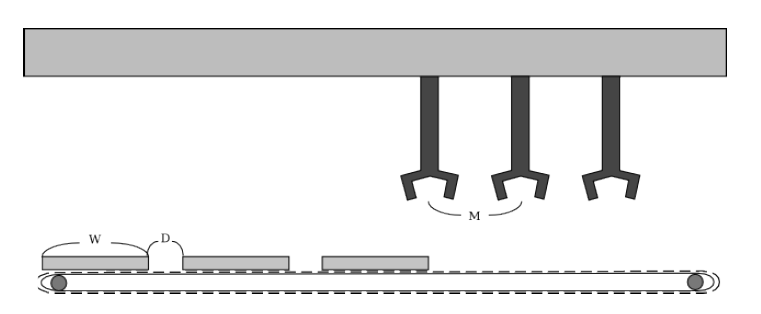Time Limit : sec, Memory Limit : KB
English

## Problem I: Tampopo Machine

"Today is another day of endless, tedious work to put a tampopo on sashimi..."

Yaruo works in a sashimi (sliced raw fish) factory. His job is to put tampopos on sashimi packages everyday. Tired of this menial job, he decided to develop a tampopo machine to do the job instead of him.

The tampopo machine has the following properties. Sashimi packages are put on a conveyor belt and move from left to right. The width of a package is $W$ and the interval between two adjacent packages is $D$. The machine has $N$ magic hands placed above the conveyor belt at regular intervals of $M$. These magic hands put tampopos every $T$ seconds.

In initial state, the right end of the first package is under the leftmost magic hand. The magic hands start putting a tampopo as soon as he turns on the power of the machine. The conveyor belt moves one unit length per one second.Unfortunately, after building the machine, Yaruo noticed that there exist some packages with no tampopos. Calculate the ratio of packages with no tampopos for him.

When a magic hand puts a tampopo on the left or right end of a package, you can assume that the tampopo is on the package.

### Input

The input consists of 5 integers, $W$, $D$, $N$, $M$ and $T$ which are described in the problem statement.
($1 \leq W, D, N, M, T \leq 1,000,000,000$)

### Output

Output the ratio of packages with no tampopos in a line. The absolute error should be less than or equal to $10^{-9}$.

### Sample Input 1

1 1 1 1 1

### Sample Output 1

0.000000000000

### Sample Input 2

1 2 3 4 5

### Sample Output 2

0.200000000000

### Sample Input 3

3 2 2 1 6

### Sample Output 3

0.166666666667